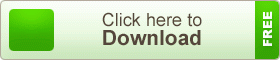# Math Solution of Electric Current & Circuit

## Math Solution of Electric Current & Circuit

Electric current is the rate of charge flow past a given point in an electric circuit, measured in Coulombs/second which is named Amperes. In most DC electric circuits, it can be assumed that the resistance to current flow is a constant so that the current in the circuit is related to voltage and resistance by Ohm’s law. The standard abbreviations for the units are 1 A = 1C/s.

An electrical circuit is a path which electrons from a voltage or current source flow. Electric current flows in a closed path called an electric circuit.

### Math Solution of Electric Current & CircuitMath Solution of Electric Current & CircuitMath Solution of Electric Current & CircuitMath Solution of Electric Current & CircuitMath Solution of Electric Current & CircuitAn electric current is a flow of electric charge. Electric charge flows when there is voltage present across a conductor.

In electric circuits this charge is often carried by moving electrons in a wire. It can also be carried by ions in an electrolyte, or by both ions and electrons such as in a plasma.

The SI unit for measuring an electric current is the ampere, which is the flow of electric charges through a surface at the rate of one coulomb per second. Electric current can be measured using an ammeter.

Electric currents cause many effects, notably heating, but also induce magnetic fields, which are widely used for motors, inductors and generators.

The conventional symbol for current is I, which originates from the French phrase intensité de courant, or in English current intensity. This phrase is frequently used when discussing the value of an electric current, but modern practice often shortens this to simply current. The I symbol was used by André-Marie Ampère, after whom the unit of electric current is named, in formulating the eponymous Ampère’s force law which he discovered in 1820. The notation travelled from France to Britain, where it became standard, although at least one journal did not change from using C to I until 1896.